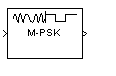# M-PSK Demodulator Baseband

Demodulate PSK-modulated data

• Library:
• Communications Toolbox / Modulation / Digital Baseband Modulation / PM

Communications Toolbox HDL Support / Modulation / PM

•## Description

The M-PSK Demodulator Baseband block demodulates a baseband representation of a PSK-modulated signal. The modulation order, M, is equivalent to the number of points in the signal constellation and is determined by the M-ary number parameter. The block accepts scalar or column vector input signals.

## Ports

### Input

expand all

Input port accepting a baseband representation of a PSK-modulated signal.

Data Types: `single` | `double` | `int8` | `int16` | `int32` | `uint8` | `uint16` | `uint32` | `Boolean`

### Output

expand all

Output signal, returned as a scalar or vector. The output is a demodulated version of the PSK-modulated signal.

Data Types: `single` | `double` | `fixed point`

## Parameters

expand all

Specify the modulation order as a positive integer power of two.

Example: `2` | `16`

Specify the elements of the input signal as integers or bits. If Output type is `Bit`, the number of samples per frame is an integer multiple of the number of bits per symbol, log2(M).

Specify the demodulator output to be hard decision, log-likelihood ratio (LLR), or approximate LLR. The LLR and approximate LLR outputs are used with error decoders that support soft-decision inputs such as a Viterbi Decoder, to achieve superior performance. This parameter is available when Output type is `Bit`.

See Phase Modulation for algorithm details. The output values for ```Log-likelihood ratio``` and ```Approximate log-likelihood ratio``` decision types are of the same data type as the input values

Specify the source of the noise variance estimate. This parameter is available when Decision type is `Log-likelihood ratio` or `Approximate log-likelihood ratio`.

• To specify the noise variance from the dialog box, select `Dialog`.

• To input the noise variance from an input port, select `Port`.

Specify the estimate of the noise variance as a positive scalar. This parameter is available when Noise variance source is `Dialog`.

This parameter is tunable in all simulation modes. If you use the Simulink® Coder™ rapid simulation (RSIM) target to build an RSIM executable, then you can tune the parameter without recompiling the model. Avoiding recompilation is useful for Monte Carlo simulations in which you run the simulation multiple times (perhaps on multiple computers) with different amounts of noise.

Note

The exact LLR algorithm computes exponentials using finite precision arithmetic. Computation of exponentials with very large positive or negative magnitudes might yield:

• `Inf` or `-Inf` if the noise variance is a very large value

• `NaN` if both the noise variance and signal power are very small values

When the output returns any of these values, try using the approximate LLR algorithm because it does not compute exponentials.

Specify how the integer or group of log2(M) bits is mapped to the corresponding symbol.

• When Constellation ordering is set to `Gray`, the output symbol is mapped to the input signal using a Gray-encoded signal constellation.

• When Constellation ordering is set to `Binary`, the modulated symbol is exp(+j2πm/M), where ϕ is the phase offset in radians, m is the integer output such that 0 ≤ m ≤ M – 1, and M is the modulation order.

• When Constellation ordering is `User-defined`, specify a vector of size M, which has unique integer values in the range [0, M–1]. The first element of this vector corresponds to the constellation point having a value of e with subsequent elements running counterclockwise.

Example: `[0 3 2 1]`

Specify the order in which input integers are mapped to output integers. The parameter is available when Constellation ordering is `User-defined`, and must be a row or column vector of size M having unique integer values in the range [0, M – 1].

The first element of this vector corresponds to the constellation point at 0 + Phase offset angle, with subsequent elements running counterclockwise. The last element corresponds to the -2π/M + Phase offset constellation point.

Specify, in radians, the phase offset of the initial constellation as a real scalar.

Example: `pi/4`

Specify the data type of the demodulated output signal.

## Block Characteristics

 Data Types `Boolean` | `double` | `fixed pointa, b, c` | `integer` | `single` Multidimensional Signals `no` Variable-Size Signals `yes` a M = 2, 4, 8 only.b Fixed-point inputs must be signed.c When ASIC/FPGA is selected in the Hardware Implementation Pane, output is ufix(1) for bit outputs, and ufix(ceil(log2(M))) for integer outputs.

## Algorithms

expand all

 Proakis, John G. Digital Communications. 4th ed. New York: McGraw Hill, 2001.